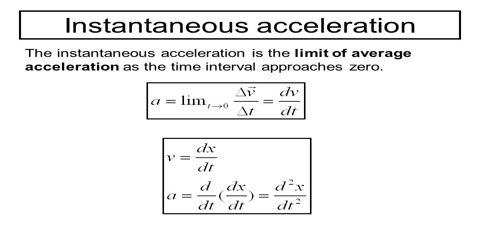# Instantaneous Acceleration related to Motion

Instantaneous acceleration: If the time interval approaches zero, the rate of change of velocity with time of a body is called the instantaneous acceleration or simply acceleration.

Explanation: Let the change of velocity at exceedingly small time interval ∆t be ∆v. Then instantaneous acceleration is obtained by dividing ∆v by the exceedingly small time during which change of velocity has taken place. The limit of a rate as the denominator approaches zero is called a derivative. Instantaneous acceleration is then the limit of average acceleration as the time interval approaches zero — or alternatively, acceleration is the derivative of velocity.So, instantaneous acceleration, a = lim∆t→0 ∆v/∆t = dv/dt

Hence, instantaneous accelerations may be defined as follows: If the time interval tends to zero, the limiting value of average acceleration is equal to the acceleration

The magnitude of acceleration is, a = │dv/dt│

It is to be mentioned that instantaneous acceleration at any point is along the perpendicular direction of the velocity of the body at that point.

Acceleration is again divided into two types: Variable acceleration and Uniform acceleration.